﻿ The Integration of Hamilton Principle with Einstein Field EquationInternational Journal of Theoretical and Mathematical Physics

p-ISSN: 2167-6844    e-ISSN: 2167-6852

2019;  9(2): 45-50

doi:10.5923/j.ijtmp.20190902.04### The Integration of Hamilton Principle with Einstein Field Equation

Ge Guangzhou

Harbin Institute of Technology, Harbin, China

Correspondence to: Ge Guangzhou, Harbin Institute of Technology, Harbin, China.
 Email: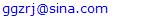Abstract

Hamilton Principle was entering into one side and Einstein Field Equation(EFE) was entering into the other side, based on the Postulate of Completeness and the relativity between reality and virtuality in physics, the author would rather integrate Hamilton Principle with EFE so as to build a new physical principle referred to as Hamilton Tensor Equation (HTE), which could substitute for Hamilton Principle and also EFE to depict the relationship among time, space, matter and energy, and thus the author deemed that it should be the virtuality of physical space rather than its curvature that determined its distribution, and further the new distribution so determined by HTE should be more complete in nature than that distribution of energy-momentum-tensor as defined by EFE. At last, the author deduced some results and findings about this new physical principle especially on connectivity and crossing of space and time.

Keywords: Hamilton Principle, Einstein Field Equation (EFE), Relativity, Completeness, Hamilton Tensor Equation (HTE)

Cite this paper: Ge Guangzhou, The Integration of Hamilton Principle with Einstein Field Equation, International Journal of Theoretical and Mathematical Physics, Vol. 9 No. 2, 2019, pp. 45-50. doi: 10.5923/j.ijtmp.20190902.04.

### 1. Introduction

The author would mainly focus on the research of fundamental issues in physics and endeavor to put forward the new physical principle through the critical thinking of existing theories. Under the guidance of this thought, the author thought that the typical theories that could interpret the relationship among time, space, matter and energy should at least include Hamilton Principle and Einstein Field Equation (EFE), among them the Hamilton Principle was established on the Principle of Least Action and EFE should have bridged the gap between space-time and matter-energy. And also the author thought this two existing theories should have depicted the relationship among time, space, matter and energy from different perspectives and levels, specifically, the Hamilton Principle should represent the law of locating the real motion path out of all the possible motion paths of the physical system and EFE definitely express the relationship between curvature of space-time and distribution of energy-momentum. However, what’s on earth the relationship among time, space, matter and energy, or exactly how were these fundamental physical quantities produced originally and interacting with each other? To answer the basic questions like this, the author didn’t believe this two theories would suffice. Thus based on this two theories and also further thinking of relativity and completeness in physics, the author further explored the relationship among time, space, matter and energy and attempted to put forward a new physical principle which could be regarded as another effort to reveal the nature of physics.

### 2. Hamilton Principle and Einstein Field Equation (EFE)

The author would at first introduce Hamilton Principle and EFE respectively as follows.
(1) Hamilton Principle
According to analytical mechanics,  Hamilton Principle is the variational principle which applies to holonomic dynamic system, that is, in the space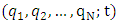of (N+1) dimensions the integral of kinetic potential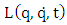of the line linking any two points to time will get the stationary value in its real motion path.
Suppose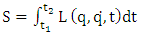(1)
S is referred to as the Hamilton Action Quantity, then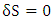(2)
And then its variational form is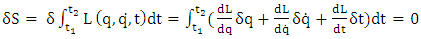(3)
And thus the dynamic problem of physical system should actually turn out to be a variational principle, that is, as far as the holonomic system is concerned, among all the possible motions of the system which are to be conditioned by the same start/finish time, the same start/finish location and the same constraints, the motion that enables Hamilton Action Quantity S to be the stationary value should be the one that really occurs in the system, and this is referred to as Hamilton Principle. Thus Hamilton Principle could be regarded as the function of energy L and time t which could depict the system’s action quantity with the integral equation and use the variational method to calculate the motion equation of overall system, and therefore Hamilton Principle should represent the law of locating the real motion path out of all the possible motion paths of the system.
(2) Einstein Field Equation (EFE)
According to the general theory of relativity, the Einstein Field Equation (EFE) may be written in the form as follows: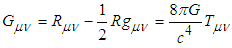(4)
where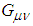is the Einstein Tensor,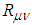is the Ricci Curvature Tensor,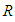is the Scalar Curvature,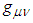is the Metric Tensor,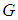is Newton's Gravitational Constant,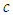is the Speed of Light in vacuum, and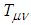is the Energy–Momentum Tensor.
The left of this equation is Einstein’s Tensor which indicates the curvature of space-time or physical space and the right side represents the distribution of matter-energy as defined by the Energy-Momentum Tensor, and it could be noted that it’s the curvature of space-time that determines the distribution of matter-energy, that is, the essential feature of general relativity is to integrate time with space into oneness so as to define the Energy-Momentum Tensor accordingly, and thus the system’s matter-energy distribution as defined by EFE could be deemed as the function of time and space or exactly that of time and coordinate.
Since then many researches have been conducted by physicists and the author would like to cite the following for further reference.
Although the Einstein Field Equations were initially formulated in the context of a four-dimensional theory, some theorists have explored their consequences in n dimensions.  And despite the simple appearance of the equations they are actually quite complicated. Given a specified distribution of matter and energy in the form of a momentum–energy tensor, the EFE are understood to be equations for the metric tensor gμν, as both the Ricci tensor and scalar curvature depend on the metric in a complicated nonlinear manner. In fact, when fully written out, the EFE are a system of ten coupled, nonlinear, hyperbolic-elliptic partial differential equations.  In addition, one can also discover new solutions of the Einstein Field Equations via the method of orthonormal frames as pioneered by Ellis and MacCallum.  In this approach, the Einstein Field Equations are reduced to a set of coupled, nonlinear, ordinary differential equations. As discussed by Hsu and Wainwright,  self-similar solutions to the Einstein Field Equations are fixed points of the resulting dynamical system. New solutions have been discovered using these methods by LeBlanc  and Kohli and Haslam. 
However, after further review the author deemed that the researches and solutions as conducted above by these theorists are not relevant to the special analysis methods to be adopted here by the author.

### 3. Methodology Support

In this part the author would conduct some further thinking on the completeness and relativity in physics, which would then be used as the theoretical preparation and methodology for the further examination of Hamilton Principle and EFE as discussed above.
(1) Postulate of Completeness
According to the Uncertainty Principle, a system’s state could be completely depicted by the function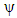of coordinate q and time t, or alternatively it could be completely depicted by the function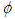of momentum p and time t, but it could not be completely depicted by coordinate q and momentum p (or energy E and time t). However, just as Einstein asserted that the depiction of state functionorshould not be complete in nature otherwise the system’s state as expressed by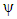orshould be certain rather than probabilistic even as far as the interpretation of an individual particle is concerned. 
After further thinking, the author asserted that a system’s state should be able to be completely depicted by Hamilton Principle as discussed above with the reason to be that this very depiction by Hamilton Principle should have covered all the possible motion paths of the system, from which the one real motion path of the system was so determined, and thus the state of the system so determined by Hamilton principle should be deterministic rather than probabilistic. Further, now that the Hamilton Principle was actually the function of energy and time, the system’s state so determined should just be able to be completely depicted by the function of energy E and time t, as would go against the Uncertainty Principle though. Thus the author deduced that the Hamilton Principle should just represent the true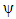or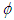as sought after by Einstein in which both energy E and time t occur. Furthermore, considering there was but the one real motion path it could be deduced that this deterministic depiction of the system was to be unique, or there was but one this deterministic depiction of system’s state otherwise the system was still to be uncertain, or in other words, the other possible depictions of system if any must be equivalent or identical with this very depiction as represented by the Hamilton Principle otherwise the system was still to be uncertain.
The author would then propose a postulate to summarize the above deductions, which could be referred to as the Postulate of Completeness, that is, a system’s state could not be completely depicted by the function of coordinate q and time t but could be completely depicted by that of energy E and time t, and in the meanwhile considering the coordinate q and momentum p were the conjugated variables about same as the energy E and time t, the author would proceed to propose another postulate, that is, a system’s state could not be completely depicted by the function of energy E and momentum p but could be completely depicted by that of coordinate q and momentum p. 
(2) Relativity between Reality and Virtuality
The author deemed that the production and interaction of fundamental quantities in physics such as time, space, matter and energy should have gone through a process of creating something from nothing if only these quantities were fundamental enough, which could be formally referred to as a process of transitioning from virtuality to reality in physics, in which the virtuality could be regarded as the existence at a higher level than that of reality. Actually considering the reality could be so produced from virtuality the author deduced that there must be certain equivalence or correspondence between reality and virtuality in physics. And thus the author would seek to identify or find out the very correspondence or equivalence between reality and virtuality in physics, which could realize the transitioning of physical system from virtuality to reality, and the author would refer to this correspondence or equivalence as the Relativity between Reality and Virtuality in physics.
Thus in the light of relativity between reality and virtuality in physics, now that the left side of EFE represented the curvature of physical space and the right side was actually the distribution of energy-momentum-tensor, the author believed EFE would to this extent represent the correspondence or relativity between reality and virtuality in physics, that is to say, the virtuality was to be expressed by curvature of physical space and the reality was to be expressed by the corresponding distribution of energy-momentum-tensor so defined.
However, it should be noted that according to the Postulate of Completeness the very distribution of energy-momentum-tensor itself should not be complete in nature because it was just the function of energy E and momentum p, and thus it should not be complete to express the reality with the distribution of energy-momentum-tensor, which would then indicate that it should not be complete to express the virtuality with the curvature of physical space.
Thus considering the incompleteness of depiction of virtuality with curvature of physical space the author deduced it should be appropriate to depict the virtuality of physical system with the Hamilton Principle which serving as a complete depiction determined the one real path out of all the possible motion paths in physical system, and in the meanwhile considering that incompleteness of depiction of reality with the distribution of energy-momentum-tensor as defined by EFE the author deemed necessary to depict the reality with some sort of more complete distribution instead.

### 4. Putting Forward a New Physical Principle

Based on the discussion above on completeness and relativity in physics, in this part the author would endeavor to put forward a new physical principle so as to completely depict the relationship among time, space, matter and energy.
(1) Hamilton Tensor Equation (HTE)
Based on the above discussion, the author would rather combine Hamilton Principle with EFE to build a new equation to completely depict the correspondence between reality and virtuality in physics. Considering the completeness of depiction by Hamilton Principle and referring to the structure format of EFE, the author would take the virtuality of physical system as the left side of the new equation which could be depicted by Hamilton Principle and in the meanwhile define the right side as the reality of physical space which could be depicted by the new distribution of energy-time-tensor just considering what Hamilton Principle depicted should actually be regarding the relationship between energy and time. Therefore, the author would endeavor to build the following expression as the equation to completely depict the correspondence or relativity between reality and virtuality in physics, that is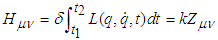(5)
where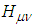is referred to as the Hamilton Tensor, which is derived from the Hamilton Principle and could represent the virtuality of physical system or physical space,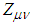is referred to as the Energy-Time-Tensor, and k is the proportional coefficient that is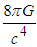as entered into the EFE. This new equation could then be referred to as Hamilton Tensor Equation (HTE).
Based on HTE the real path as determined by Hamilton Principle would turn out to be the physical space as determined by this distribution of energy-time-tensor as defined by HTE, and the real path or the physical space so determined should not be affected by or subject to any forces due to its own completeness. And further considering this system of physical space should contain countless possible motion paths as depicted by Hamilton Principle, it should permit the phenomenon of co-occurrence at different locations in the physical system, which meant the proper velocity of the system might break through the limitation of speed of light. Therefore, just like the circumstance as depicted by EFE where an object would not be subject to the gravitational force in an appropriate physical space, an object would not be subject to any forces or even the limitation of speed of light and would rather conduct a free motion in the physical space as determined by the distribution of energy-time-tensor as defined by HTE.
(2) Completion of Hamilton Tensor Equation (HTE)
At first, now that what the Lagrangian function L represented should actually be energy, the author might as well substitute the energy E for the Lagrangian function L so that a more generalized format of HTE could be formulated, that is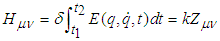(6)
The physical meaning of this equation (6) is that the virtuality of physical space should determine the corresponding distribution of energy-time-tensor.
Further considering momentum p and coordinate q are about the same conjugated variables as energy E and time t, the author would then formulate another Hamilton Tensor Equation (HTE), that is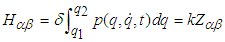(7)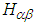on the left of the equation represents the virtuality of physical space as well, which is the Hamilton Tensor as formulated by momentum p and coordinate q,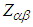on the right side represents the distribution of momentum-coordinate-tensor, and k is the same proportional coefficient. The physical meaning of this equation (7) is that the virtuality of physical space should determine the corresponding distribution of momentum-coordinate-tensor.
The author believed the conjugated equations (6) and (7) should constitute the complete form of HTE. According to this two equations, the real path as determined by Hamilton Principle would in nature be either the physical space as determined by the distribution of energy-time-tensor as defined by HTE (6) or the physical space as determined by the distribution of momentum-coordinate-tensor as defined by HTE (7), and further this two equations should be equivalent to each other serving as the complete depiction of physical system.

### 5. Results and Findings

(1) Connectivity of Space and Time
At first, now that the real path as determined by the equation (6) would not be subject to any forces or even limitations of speed of light, and also considering this real path should actually be determined by the corresponding distribution of energy-time-tensor, the author deduced that the free motion in space or the correlation of faster-than-light speed or even the instant connection of space could be realized on this real path, and this real path should just be the physical space as determined by the distribution of energy-time-tensor to be obtained by solving the equation (6).
On the other hand, now that the real path as determined by the equation (7) would not be subject to any forces or even limitations of speed of light, and also considering this real path should actually be determined by the corresponding distribution of momentum-coordinates-tensor, the author deduced that the free motion in time or the correlation of faster-than-light speed or even the coexisting connection of time could be realized on this real path, that is to say, on this real path the free motion in time could be realized without any restrictions and the space could then connect time in a coexisting way and thus the past, now and future could coexist at one same location in space, and this real path should just be the physical space as determined by the distribution of momentum-coordinates-tensor to be obtained by solving the equation (7).
Therefore the author deduced that the connectivity of space and time could be so realized as discussed above.
(2) Traveling Across Space and Time
In the physical system as depicted by Hamilton Principle, an object would not be subject to any forces or even limitations of speed of light but conduct a free motion on the real path which is just the physical space as determined by HTE. The author thought HTE could then be used as a new dynamic principle for traveling across space and time.
The author then deduced that a spacecraft would be able to travel freely in space at a faster-than-light speed if it flied on the real path as determined by the equation (6), and thus the interplanetary flight even beyond the solar system could possibly be realized in a short time. Specifically to make this true, at first it needs to carve out a new route for the spacecraft to fly from the earth to the target planet in space, and then according to the equation (6) the real path should be determined as the route for the spacecraft to follow, and thus the spacecraft would not be restricted by any forces or speed of light but travel in space freely if only it followed the real path, which should be able to be implemented by the application of automatic navigating. In addition it should be noted that the real path so determined would not be restricted by the gravitational force as it would not be restricted by any forces or even speed of light as discussed above, thus the spacecraft following the real path would not be restricted by the gravitational force either, that is to say, the spacecraft following the real path would not be restricted by the first and the second and even the third cosmic velocities, which means the spacecraft could possibly fly into space unrestrictedly with no need for the powerful thrust as equipped and provided by the power units such as rockets. Therefore the spacecraft could theoretically travel faster than light on the real path and even arrive at any destination planets instantly as the real path could realize the instant connection of space, and thus the author deduced that the interplanetary travel could be achieved in a comparatively short time hopefully.
Likewise, referring to the depiction above about the spacecraft traveling freely in space, a spacecraft would also be able to travel freely and unrestrictedly in time without limitations by any forces or even speed of light if only it followed the real path as determined by the equation (7), that is to say, the spacecraft could fly to the past or the future from now due to the coexisting connection of time so that the free motion in time at one same location could be achieved.
Based on the above discussion it seemed to be viable for the spacecraft to travel across space and time freely with no restrictions.
(3) Reinterpretation of Quantum Entanglement - Communication Crossing Space and Time
As a phenomenon of quantum mechanics, the quantum entanglement was a kind of wave as originally proposed by Einstein, Podolsky and Rosen. Specifically, the quantum entanglement was the phenomenon that one particle would interfere with another particle in the system comprising two or more particles although the particles involved might be quite apart from each other in space. That is to say, the state of one particle would influence that of another particle even though they might be far away from each other, or in other words, if one particle was somehow manipulated such as by a quantum measurement and changed its state, another particle would at once change its state accordingly. Einstein referred to the quantum entanglement as spooky action at a distance. 
Based on above description of quantum entanglement and pursuing understanding of Hamilton Tensor Equation (HTE), the author would assume that the quantum entanglement to be actually the phenomenon that one particle would interfere with another particle in the system comprising two or more particles located on one same real path as discussed above. Specifically, now that an object would not be restricted by any forces or even speed of light but conduct the free motion in the appropriate physical space which was the real path as determined by HTE, the very free motion conducted on the real path would realize the instant connection of space or the coexisting connection of time as depicted above and thus the two or more particles which were located on one same real path would so correlate with each other accordingly. That is to say, when a particle on a real path was somehow manipulated such as by a quantum measurement the whole real path or the corresponding physical space where the particle was located would be so manipulated and thus all the particles which were located on this same real path would be so influenced and manipulated accordingly, as could be referred to as the real-path-correlation which the author believed to be the mechanism of quantum entanglement.
Furthermore based on above discussion it should be noted that the real path itself or this very real-path-correlation serving as the mechanism of quantum entanglement would not be restricted by any forces. As a matter of fact, now that the quantum entanglement might occur at a distance beyond the scope of gravitational force of the system, which could be verified by some experiments conducted so far, this should indicate that the quantum entanglement would at least not be restricted or influenced by the gravitational force.
According to the mechanism of quantum entanglement, if a signal were to be transmitted along the very real path as determined by HTE the instant connection of space or coexisting connection of time could be realized accordingly, and thus the long distance interplanetary communication crossing space could be so achieved and also the communication crossing time would become possible, and further considering the characteristics of real-path-correlation, the infrastructure such as base stations and satellites which were required for normal signal transmitting might not be so necessary.

### 6. Conclusions

This article could be regarded as a bold exploration of fundamental physical principles. Pursuing understanding of completeness and relativity in physics, the author deemed that both Hamilton Principle and EFE which depict the relationship among matter, energy, time and space were to be problematic, and thus the author proposed to integrate Hamilton Principle with EFE so as to build a new physical principle which could be referred to as Hamilton Tensor Equation (HTE) and could depict the very relativity between reality and virtuality in physics and also could completely depict the distribution of physical space. The author would indicate the significance of the work as highlighted below:
• Postulate of Completeness;
• Relativity between reality and virtuality;
• Integration of Hamilton approach with EFE, which deduced that it should be the virtuality of physical space rather than its curvature that determines its distribution;
• Connectivity of space and time;
• Reinterpretation of quantum entanglement;
• Traveling and communicating across space and time.

### ACKNOWLEDGMENTS

The author gratefully acknowledge the support of Harbin Institute of Technology, and the author would also like to thank the referee for his/her helpful suggestions upon reviewing this paper.

### References

  Analytical Mechanics, L.N. Hand, J.D. Finch, Cambridge University Press, 2008, ISBN 978-0-521-57572-0.  Einstein A. (1916), Relativity: The Special and General Theory (Translation 1920), New York: H. Holt and Company.  Grøn, Øyvind; Hervik, Sigbjorn (2007). Einstein's General Theory of Relativity: With Modern Applications in Cosmology (illustrated ed.). Springer Science & Business Media. p. 180. ISBN 978-0-387-69200-5.  Stephani, Hans; Kramer, D.; MacCallum, M.; Hoenselaers, C.; Herlt, E. (2003). Exact Solutions of Einstein's Field Equations. Cambridge University Press. ISBN 0-521-46136-7.  Rendall, Alan D. "Theorems on existence and global dynamics for the Einstein equations." Living Reviews in Relativity 8.1 (2005): 6.  Ellis, G. F. R.; MacCallum, M. (1969). "A class of homogeneous cosmological models". Comm. Math. Phys. 12 (2): 108–141.  Hsu, L.; Wainwright, J (1986). "Self-similar spatially homogeneous cosmologies: orthogonal perfect fluid and vacuum solutions". Class. Quantum Grav. 3: 1105–1124.  LeBlanc, V. G. (1997). "Asymptotic states of magnetic Bianchi I cosmologies". Class. Quantum Grav. 14: 2281.  Kohli, Ikjyot Singh; Haslam, Michael C. (2013). "Dynamical systems approach to a Bianchi type I viscous magnetohydrodynamic model". Phys. Rev. D. 88: 063518.  Physics: Its development and philosophy, Ta-You Wu, Int. J. Mod. Phys. A, 04, 4643 (1989).  Ge Guangzhou. “The Discussion on the Consistency of General Relativity Theory and Uncertainty Principle and Putting Forward of a New Field Equation”. International Journal of Pure and Applied Physics. ISSN 0973-1776 Volume 14, Number 1 (2018), pp. 1-13.  Einstein A, Podolsky B, Rosen N. “Can Quantum-Mechanical Description of Physical Reality Be Considered Complete?”. Phys. Rev. 47 (10): 777–780.